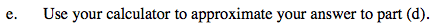### Home > PC > Chapter 6 > Lesson 6.2.3 > Problem6-95

6-95.
1. Suppose α is an angle in Quadrant I and β is an angle in Quadrant IV such that sin α =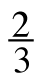and cos β =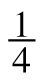. Homework Help ✎

1. Find the exact value of cos α.

2. Find the exact value of sin β.

3. Find the exact value of sin(α + β).

4. Find the exact value of cos(α + β).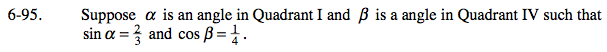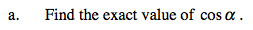Draw diagrams. Find the 3rd side for each triangle.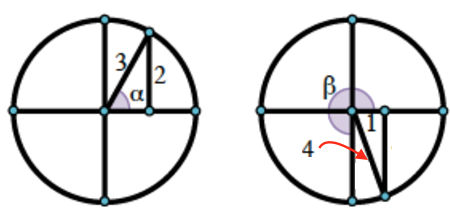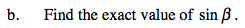Draw diagrams. Find the 3rd side for each triangle.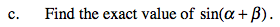Use the angle sum and difference formula for the sine.

$\frac{2-5\sqrt{3}}{12}$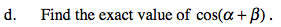Use the angle sum and difference formula for the cosine.